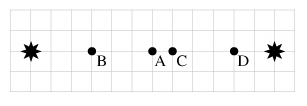# Problem: Two sources of coherent radio waves broadcasting in phase are located as shown below. Each grid square is 0.5 m square, and the radio sources broadcast at λ = 2.0 m.1. At Point A, is the interference between the two sources constructive or destructive?a) constructiveb) destructive 2. At Point B is the interference between the two sources constructive or destructive?a) constructiveb) destructive 3. At Point C is the interference between the two sources constructive or destructive?a) constructiveb) destructive 4. At Point D is the interference between the two sources constructive or destructive?a) constructiveb) destructive

###### FREE Expert Solution

Interference condition:

$\overline{){\mathbf{∆}}{\mathbf{L}}{\mathbf{=}}{\mathbf{n}}{\mathbf{\lambda }}}$

Constructive interference occurs when the path difference between the two waves is an integral multiple of the wavelength. That is, when ΔL = mλ.

Destructive interference occurs when the path difference is ΔL = (m + a)λ.

In this problem, a = 0.5.

Replace n with m and solve:

Solving for m,

$\overline{){\mathbit{m}}{\mathbf{=}}\frac{\mathbf{∆}\mathbf{L}}{\mathbf{\lambda }}}$

1.

LA1 = (6)(0.5) = 3 m (Distance from point A to the left source)

LA2 = (6)(0.5) = 3 m (Distance from A to the source in the right).

83% (104 ratings)###### Problem Details

Two sources of coherent radio waves broadcasting in phase are located as shown below. Each grid square is 0.5 m square, and the radio sources broadcast at λ = 2.0 m.1. At Point A, is the interference between the two sources constructive or destructive?

a) constructive

b) destructive

2. At Point B is the interference between the two sources constructive or destructive?

a) constructive

b) destructive

3. At Point C is the interference between the two sources constructive or destructive?

a) constructive

b) destructive

4. At Point D is the interference between the two sources constructive or destructive?

a) constructive

b) destructive